Connection is splitting of first-order symbol sequence

Statement

Suppose$E$ is a vector bundle over a differential manifold$M$. Denote by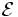$\mathcal{E}$ the sheaf of sections of$E$. Consider the first-order symbol sequence for$E$, given by: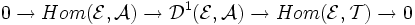$0 \to Hom(\mathcal{E},\mathcal{A}) \to \mathcal{D}^1(\mathcal{E},\mathcal{A}) \to Hom(\mathcal{E},\mathcal{T}) \to 0$

Here$\mathcal{A}$ is the sheaf of infinitely differentiable functions on$M$,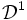$\mathcal{D}^1$ denotes the space of first-order differential operators from$\mathcal{E}$ to$\mathcal{A}$, and$\mathcal{T}$ denotes the sheaf of derivations of$M$.

A connection on$E$ is equivalent to a choice of splitting for this sequence.

Proof

A splitting of the above sequence is equivalent to a section map:$Hom(\mathcal{E},\mathcal{T}) \to \mathcal{D}^1(\mathcal{E},A)$

which is equivalent to a map (satisfying some additional conditions):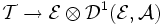$\mathcal{T} \to \mathcal{E} \otimes \mathcal{D}^1(\mathcal{E},\mathcal{A})$

The right side is equivalent to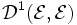$\mathcal{D}^1(\mathcal{E},\mathcal{E})$, so a splitting of the sequence is equivalent to a map: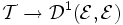$\mathcal{T} \to \mathcal{D}^1(\mathcal{E},\mathcal{E})$

satisfying some additional conditions. Clearly, a connection is also a map of the above form, so it remains to check that the additional condition that comes from it being a splitting, is equivalent to the Leibniz rule for the connection.